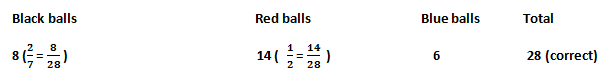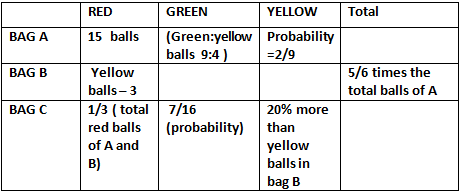# Probability Questions Based on SBI PO Mains 2017#### Question 1:

A bag contains some Black balls, Red balls and Blue balls such that the probability of picking a Black ball is 2/7 and the probability of picking a Red ball is ½. If the number of Blue balls are 6.Find the total number of balls ? (SBI PO MAINS 31-07-2016

(a) 23
(b) 24
(c) 14
(d) 28
(e) none of the these

#### Question 2

A bag contains some Black balls, Red balls and Blue balls such that the probability of picking a Black ball is 2/7 and the probability of picking a Red ball is ½.  If all the Balls are numbered starting from 1,2, 3, …. And so on , then what is the probability of getting one ball numbered as multiple of 3 or 7 out of total balls.

(a) 3/7
(b) 5/14
(c) 1/12
(d) 6/11
(e)none of these

#### Question 3

There are three bags A, B and C filled with balls of three different colours i.e. Red, Green and Yellow colour. Bag A has 15 red balls .The probability of drawing a Yellow ball out of the Bag A is 2/9. The ratio of the number of Green and Yellow balls in Bag A is 9:4. The number of total balls in Bag B is 5/6 times the total number of balls in Bag A. The number of red balls, however, is 3 less than the number of Yellow balls .The number of Green balls is 2/3 times the number of green balls in Bag A.
The number of Red balls in Bag C is one-third of the total number of Red balls in both A and B together. The number of Yellow balls is 20% more than the number of yellow balls in bag B.The probability of drawing a green ball from bag C is 7 /16.
“P” Yellow balls are now transferred from Bag A to bag C and now the probability of drawing a yellow ball from bag C is 2/5. Find the value of P.

(a) 2
( b) 3
(c) 13
(d) 27
(e) None of these

#### Question 4:

There are three bags A, B and C filled with balls of three different colours i.e. Red, Green and Yellow color. Bag A has 15 red balls. The probability of drawing a Yellow ball out of the Bag A is 2/9.The ratio of the number of Green and Yellow balls in Bag A is 9:4. The number of total balls in Bag B is 5/6 times the total number of balls in Bag A. The number of red balls, however, is 3 less than the number of Yellow balls and the number of Green balls is 2/3 times the number of green balls in Bag A. The number of Red balls in Bag C is one-third of the total number of Red balls in both A and B together. The number of Yellow balls is 20% more than the number of yellow balls in bag B.The probability of drawing a green ball from bag C is 7 /16. If one ball is drawn at random from any one of the bag. Find the probability that the drawn ball is yellow in colour?

(a) 57/216
(b) 67/216
(c) 77/216
(d) 87/216
(e) None of these

#### Solution (1)

This can be solved by trial and error method
You know well the probability of getting black balls is 2/7 and of red balls is 1/2 , also Number of blue balls is 6.Now the total number of balls will be a multiple of both 7 and 2 i.e. it can be 14 , 28 , etc. Suppose if we take total number of balls to be 14 and try distributing accordingly, we getSuppose if we take total number of balls to be 28 and try distributing accordingly, we get(1) So 28 is the total number of balls (D)

#### Solution (2)

21 is the only number which is multiple of both 3 and 7, so while calculating probability separately for 3 and 7, this number 21 will be taken twice so to avoid confusion we take it once here and will exclude 21 while calculating probability separately. Also, number 21 can only be taken once.
➡ Probability of getting number multiple of both 3 and 7 (number 21) =1/28
➡ Probability of getting numbers which are multiple of 3 ( 3,6,9,12,15,18,24,27) = 9 / 28
➡ Probability of getting numbers which are multiple of 7 (7,14,28) =3/28
➡ Probability of getting a number either multiple of 3 or 7 = 3/28 + 9/28 + 1/ 28 = 12/28 = 3/7
Hence 3/7 (a) is the right answer.

#### Solution 3

This can also be done on trial and error method
GivenBEGIN WITH BAG A, we know Yellow bags probability is 2/9 means total balls will be a multiple of 9 ( 9, 18, 27, 36, 45, 54, 63…..). 9, 18 cannot be total number of balls
Suppose total number balls is 27 then red balls are 15, yellow balls will be 6 and green balls will be 6.the ratio of green and yellow balls must be 9:4 , this condition is not satisfied. Same way even for 36 , 45 …. Not agreeable.
• If the total number of balls taken to be 54.
• Then Red balls = 15
• Yellow balls = 12
• Green balls = 27 (green and yellow ratio 27:12 = 9:4 satisifed )
• Therefore the total number of balls is 54 in bag A.
• Now using this total number balls calculate the values for bag B i.e. given 2/3 times green balls in bag A = 2/3 *27 = 18 . Also total number of balls in Bag B is 5/6 times of toal balls of A = 54*5/6 = 45
• Now red balls are 3 less the yellow balls .let yellow balls be X and red be x-3
• 45- 18= 27
• 27 is both red plus yellow balls i.e. x + ( x-30) = 27
• X=15
• Also red balls in bag C 1/3 ( total red balls in A and B) = 1/3 ( 27)= 9
• Also 20% more green balls than B’s green bag i.e. 15*120/100 = 18
• And using probability 9/16 remaining data is foundif p yellow balls are removed from bag A and transferred to bag C then
18+ p / 48+ p = 2/5
Solving this we get 2. Hence (a)

#### Solution (4)

Only yellow balls have to be chosen from any of the bags
Firstly in bag A you have to choose yellow ball out of 3colors so 1/3, then out of 12/54
Similarly for other two bags
1/3 ( 12/54) + 1/3 ( 15/45) + 1/3 ( 18/48)
1/3( 12/54 + 15/45 + 18/48) = 1/3(67/216)
Hence (b)#### What's trending in BankExamsToday

Smart Prep Kit for Banking Exams by Ramandeep Singh - Download here